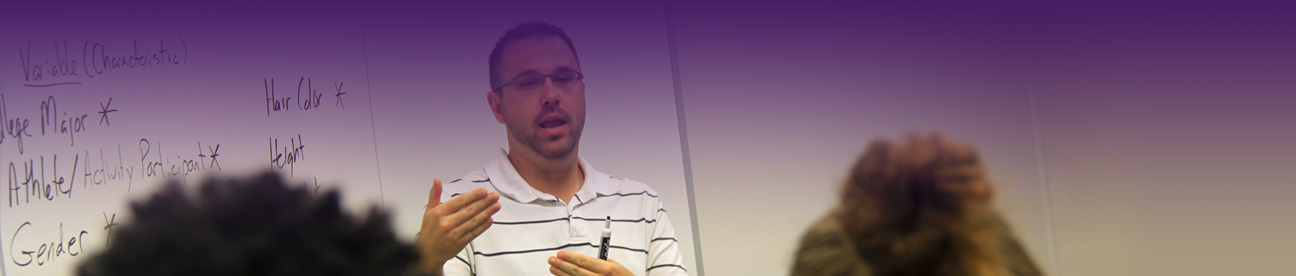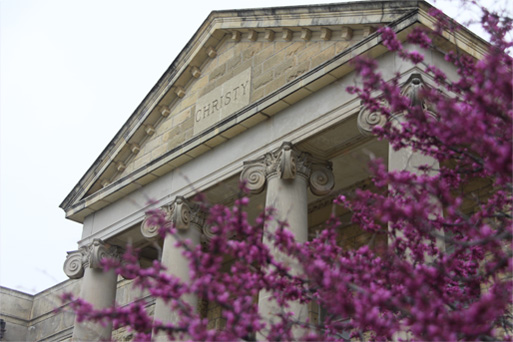Course Descriptions

MATH099 Elementary Algebra
This course is designed to provide a thorough study in the arithmetic of whole numbers, fractions with elementary applications in consumer math and measurement and introduce students to the basic concepts of algebra. Students that successfully complete this course should be prepared to enroll in MATH105. (Three credits of enrollment credit. These hours will not count toward graduation.)

MATH103 Foundations of Basic Mathematics
Numbers and operations, fractions and operations, decimals, ratio, proportion and percent, introduction to algebra, geometry, measurement, statistics, and problem-solving techniques, including the use of technology. Credit 3 hours.

MATH105 Intermediate Algebra
Fundamental operations, factoring, fractions, positive, negative, zero, and fractional exponents, linear and quadratic equations, systems of linear equations. Students wanting to enroll in this course should normally have successfully completed one year of high school algebra. Questions concerning the adequacy of a student’s preparation for this course should be directed to a member of the mathematics department. Credit 3 hours.

MATH110 College Algebra
A review of Intermediate Algebra with more depth in the topics. Also logarithmic and exponential functions, the binomial theorem, systems of quadratic equations, and matrices and determinants. Prerequisites: MATH 105 with a grade of C- or better or consent of instructor. Credit 3 hours.

MATH112 Trigonometry
A basic course in plane trigonometry. Prerequisite: MATH 110 or consent of instructor. Credit 3 hours.

MATH215 Introduction to Statistics and Probability
An introduction to statistics and probability with emphasis on applications. Topics include numerical and graphical displays of data, linear regression, hypothesis testing, and elementary probability, including counting methods, conditional probabilities, and probability distributions. Prerequisite: MATH 105 with a grade of C- or better or consent of instructor. Credit 3 hours.

MATH222 Calculus 1
Limits, differentiation, and antidifferentiation of elementary and transcendental functions with applications. Prerequisite: MATH110 with a grade of C- or better or consent of instructor. Credit 4 hours.

MATH223 Calculus 2
Techniques and applications of integration, sequences and series, and a brief introduction to differential equations. Prerequisites: MATH 222 with a grade of C- or better or consent of instructor. Credit 4 hours.

MATH224 Calculus 3
Vector Calculus, partial differentiation and iterative integrals with applications. Prerequisite: MATH 223 with a grade of C- or better or consent of instructor. Credit 4 hours.

MATH319 Linear Algebra
A study of matrices, determinants, vector spaces, changes in bases, and linear transformations with applications. Maple computer applications in different topics. Prerequisite: MATH 110 with a grade of C- or better or consent of instructor. Credit 3 hours.

MATH320 Introduction to Advanced Mathematics
Develops the concept of theorem proving. Covers induction, fundamentals of set theory and logic, cardinal numbers, partially ordered sets, relations, and functions. Introduction to basic structures, to algebra and analysis, and to the history of their development. Prerequisite: MATH 222 with a grade of C- or better. Credit 3 hours.

MATH321 Ordinary Differential Equations
Solution methods for ordinary differential equations, including series techniques and Laplace transforms, with applications. Prerequisite: MATH 224 with a grade of C- or better. Credit 3 hours.

MATH331 Foundations of Geometry
A study of axiomatic systems, Euclidean geometry (with emphasis on what should be taught in high school), non-Euclidean geometries, and the history of mathematics. Prerequisite: MATH 320 with a grade of C- or better. Credit 3 hours.

MATH353 Problems in Mathematical Science
Students who have demonstrated superior achievement in mathematics, by approval of the mathematics program, may enroll for independent study under the supervision of a faculty in this program. Prerequisite: MATH 320 with a grade of C- or better. Credit 1 to 3 hours.

MATH444 Abstract Algebra
Study of the classical algebraic systems. Topics include groups, symmetric groups, rings, ideals, quotient rings, and fields. Prerequisite: MATH 320 with a grade of C- or better. Credit 3 hours.

MATH445 Mathematical Analysis
Introduction to the topology of the real line, and to open and closed sets. It includes results concerning functions of one variable, limits of sequences, completeness, compactness, and continuity. Prerequisite: MATH 320 with a grade of C- or better. Credit 3 hours.

MATH447 Complex Variables
Study of the algebraic properties of complex numbers, complex functions, analytic and harmonic functions, complex integration, and series representations. Prerequisite: MATH 320 with a grade of C or better. Credit 3 hours.Build an education.

Academic excellence sets SC apart.

41 main campus and 16 professional studies undergraduate majors, 27 minors, teacher certification in 13 areas, and 6 graduate programs.